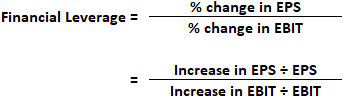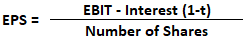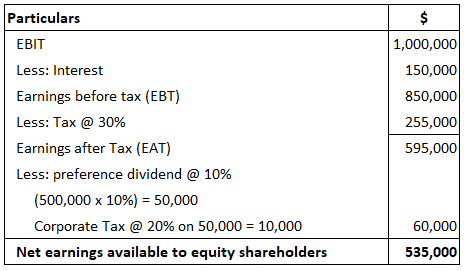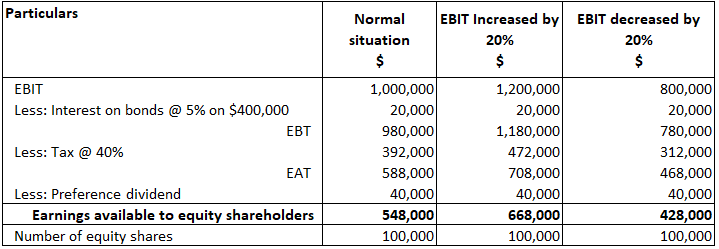## What Is Financial Leverage?

Just as operating leverage results from the existence of operating expenses in the enterprise’s income stream, financial leverage results from the presence of fixed financial charges in the firm’s income stream.

In fact, financial leverage relates to financing activities (i.e., the cost of raising funds from different sources carrying fixed charges or not involving fixed charges).

For example, if funds are raised through long-term debts such as bonds and debentures, these instruments carry fixed charges in the form of interest. This must be paid irrespective of the operating profits.

In contrast, if funds are raised through equity shares, then the dividend to be paid is not a fixed charge. Furthermore, it is paid out of operating profits.

If the funds are raised by preference shares, despite not carrying a fixed interest charge, they carry the fixed dividend rate.

Therefore, the dividend payable to preference shareholders is regarded as a fixed charge when calculating financial leverage.

It should be noted that equity shareholders are entitled to the remainder of the operating profits of the firm after meeting all the prior obligations.

Thus, financial leverage measures the relationship between the operating profit (EBIT) and earning per share (EPS) to equity shareholders. It is calculated as the percentage change in EPS divided by a percentage change in EBIT.

When calculating financial leverage, you should note that EBIT is a dependent variable that is determined by the level of EPS.

## Formula For Financial LeverageTo calculate both operating leverage and financial leverage, EBIT is referred to as the linking point in the study of leverage. When calculating the operating leverage, EBIT is a dependant variable that is determined by the level of sales.

When calculating financial leverage, EBIT is no doubt a dependant variable, but it is determined by the level of EPS. In fact, EPS is calculated using the formula below:## How to Calculate Degree of Financial Leverage

To calculate the degree of financial leverage, let’s consider an example.

XYZ Company has an EBIT of \$1,000,000.

The interest liability is \$150,000. The company has issued 10% preference shares of \$500,000 and 50,000 equity shares of \$100 each. The average tax applicable to the company is 30% and corporate dividend tax is 20%.

Required: Calculate the degree of financial leverage.

### SolutionNumber of equity shares = 50,000
EPS = Earnings available to equity holders / Number of shares = 535,000 / 50,000
EPS = 10.7
Financial leverage = EBIT / EBT -D ÷ (1 – t)
= 1,000,000 / 850,000 – 60,000 ÷ (1 – 0.30)
= 1,000,000 / 850,000 – (60,000 ÷ 0.7)
= 1.000.000 / 850,000 – 85,714
Therefore, the degree of financial leverage = 1,000,000 / 764,286 = 1.308

## Example

Let’s examine how EPS varies at different levels of EBIT, taking into account the following:

1. Actual level
2. Percentage increase in EBIT
3. Percentage decrease in EBIT

In this example, suppose that HT Limited’s EBIT for the current year is \$1,000,000. The company has 5% bonds amounting to \$400,000. What is the EPS? Suppose the EBIT is:

1. \$1,200,000
2. \$800,000

How would this affect EPS? You can assume that the company falls under the tax bracket of 40%. The number of outstanding equity shares is 100,000.

Required: Calculate the financial leverage and interpret the results.

### Solution### Interpretation

EPS = Earnings available to equity holders / Number of shares
= 548,000 / 100,000 = 5.48
= 668,000 / 100,000 = 6.68
= 428,000 / 100,000 = 4.28

1. When EBIT is raised from \$1,000,000 to \$1,200,000 with an increase of 20%, it results in a 21.90% increase in EPS, i.e., increase from 5.48 to 6.68 (6.68 – 5.48 = 1.20 ÷ 5.48 x 100)
2. When EBIT is decreased from \$1,000,000 to \$800,000 with a decrease of 20%, it results in a 21.90% decrease in EPS, i.e., decrease from 5.48 to 4.28 (5.48 -4.28 = 1.20 ÷ 5.48 x100)

Based on calculations like those shown above, the finance manager can make appropriate decisions by comparing the cost of debt financing to the average return on investment.

1. If ROI is more than the cost of debt financing, then it is called favorable financial leverage or trading on equity or positive financial leverage. This situation encourages the finance manager to go in for more and more debt financing to enhance the benefits to shareholders.
2. If ROI is less than the cost of debt financing, it is not worthwhile to seek debt financing because losses get increased and the benefits to shareholders get decreased. This situation is called unfavorable financial leverage or negative financial leverage.
3. If ROI is equal to the cost of debt financing, it is not advisable to borrow funds because the company may not be able to generate surplus earnings by debt financing.

To conclude, financial leverage emerges as a result of fixed financial cost (interest on debentures and bonds + preference dividend). If the financial leverage is positive, the finance manager can try to increase the debt to enhance benefits to shareholders.

Where earnings are either equal to fixed financial charge or unfavorable, debt financing should not be encouraged.

## Operating and Financial Leverage Viewed Together

Operating leverage helps to determine the reasonable level of fixed costs, whereas financial leverage helps to ascertain the extent of debt financing.

Both financial and operating leverage emerge from the base of fixed costs. That’s to say, operating leverage appears where there is a fixed financial charge (interest on debt and preference dividend).

The variability of sales level (operating leverage) or due to fixed financing cost affects the level of EPS (financial leverage).

It is observed that debt financing is cheaper compared to equity financing. This encourages finance managers to opt for more debt financing.

Simultaneously, one should be conscious of the risks involved in increasing debt financing, including the risk of bankruptcy. Therefore, it is suggested to have a trade-off between debt and equity so that the shareholders’ interest is not affected adversely.

As this discussion indicates, both operating and financial leverage (FL) are related to each other. Both of them, when taken together, multiply and magnify the effect of change in sales level on the EPS.

However, operating leverage directly influences the sales level and is called first-order leverage, whereas FL indirectly influences sales and is called second-order leverage. If the operating leverage explains business risk, then FL explains financial risk.

In conclusion, the higher the operating leverage, the more the company’s income is influenced by fluctuations in sales volume. If the sales volume is significant, it is beneficial to invest in securities bearing the fixed cost.

In the case of FL, the higher the amount of debt, the higher the FL. High leverage may be beneficial in boom periods because cash flow might be sufficient. During times of recession, however, it may cause serious cash flow problems.

This is because there may not be enough sales revenue to cover the interest payments.

However, the finance manager should carefully consider the situation and make a decision that enhances the benefits to shareholders. To cover the total risk and to be precise in their decision, the financial manager may rely on combined leverage.

### What is financial leverage?

Financial leverage is the use of debt to increase investment returns.

### What are the benefits and risks involved in using financial leverage?

Benefits of financial leverage include that it increases profits without increasing sales, it reduces risk on investments, and decisions can be made rapidly with less time required for planning and implementation than other methods. Some risk factors of financial leverage include interest expenses, potential bankruptcy if interests can not be paid, and loss in market value for publicly traded stock.

### What are some examples of how financial leverage can be used?

Examples of financial leverage usage include using debt to buy a house, borrowing money from the bank to start a store and bonds issued by companies.

### How is financial leverage measured?

Financial leverage is calculated using the following formula: assets ÷ shareholders' equity = debt ratio.

### How are the concepts of financial leverage and Operating Leverage related?

Financial leverage relates to Operating Leverage, which uses fixed costs to measure risk, by adding market volatility into the equation. First-order operational leverage affects income directly, whereas second-order or combined leverage affects income indirectly through fluctuations in asset values.

True is a Certified Educator in Personal Finance (CEPF®), a member of the Society for Advancing Business Editing and Writing, contributes to his financial education site, Finance Strategists, and has spoken to various financial communities such as the CFA Institute, as well as university students like his Alma mater, Biola University, where he received a bachelor of science in business and data analytics.

To learn more about True, visit his personal website, view his author profile on Amazon, his interview on CBS, or check out his speaker profile on the CFA Institute website.